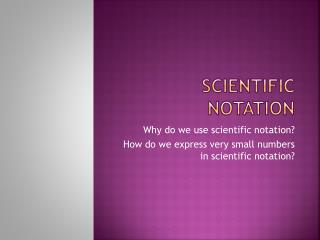# Scientific notation - PowerPoint PPT PresentationDownload PresentationScientific notation

Scientific notation
Download Presentation## Scientific notation

- - - - - - - - - - - - - - - - - - - - - - - - - - - E N D - - - - - - - - - - - - - - - - - - - - - - - - - - -
##### Presentation Transcript

1. Scientific notation Why do we use scientific notation? How do we express very small numbers in scientific notation?

2. Scientific Notation A number is expressed in scientific notation when it is in the form a x 10n where a is between 1 and 10 and n is an integer

3. Write the width of the universe in scientific notation. 210,000,000,000,000,000,000,000 miles Where is the decimal point now? After the last zero. Where would you put the decimal to make this number be between 1 and 10? Between the 2 and the 1

4. 2.10,000,000,000,000,000,000,000. How many decimal places did you move the decimal? 23 When the original number is more than 1, the exponent is positive. The answer in scientific notation is 2.1 x 1023

5. 1) Express 0.0000000902 in scientific notation. Where would the decimal go to make the number be between 1 and 10? 9.02 The decimal was moved how many places? 8 When the original number is less than 1, the exponent is negative. 9.02 x 10-8

6. Write 28750.9 in scientific notation. • 2.87509 x 10-5 • 2.87509 x 10-4 • 2.87509 x 104 • 2.87509 x 105

7. 2) Express 1.8 x 10-4 in decimal notation. 0.00018 3) Express 4.58 x 106 in decimal notation. 4,580,000

8. Turn each number into scientific notation • Dogs have about 220,000,000 olfactory receptors to help them smell—roughly 40 times the number humans have. • The population of Tokyo, Japan was approximately 34,450,000 in 2000. • The biggest iceberg ever seen, known as B-15, weighed an estimated 4,000,000,000,000 tons. • One light-year is the distance light travels in one year—about 5,900,000,000,000 miles. • Scientists discovered a black hole at the center of M87, a galaxy in the constellation Virgo, rotating at 1,200,000 miles per hour using the Hubble Space Telescope. • The planet Mercury travels at 107,000 miles per hour. • Microscopic quantities of liquid water were found trapped in salt crystals in a 4,500,000,000-year-old meteorite that fell to Earth at Monahans, Texas in 1998. • In the mid 1990’s, the world had an estimated 19,200,000 camels, of which nearly half were in Somalia and Sudan. • In the early 1990’s, Utah’s chicken population produced approximately 456,000,000 eggs. • During his lifetime, George Eastman (1854-1932), an American inventor of films and cameras, donated \$75,000,000 to charities.

9. Activity • Each of you will receive a 3 x 5 index card with a different number written in scientific notation. 3-5 students at a time come to the front of the room with their cards and have stand in order from least to greatest.

10. Googol & googolplex

11. Powers of ten